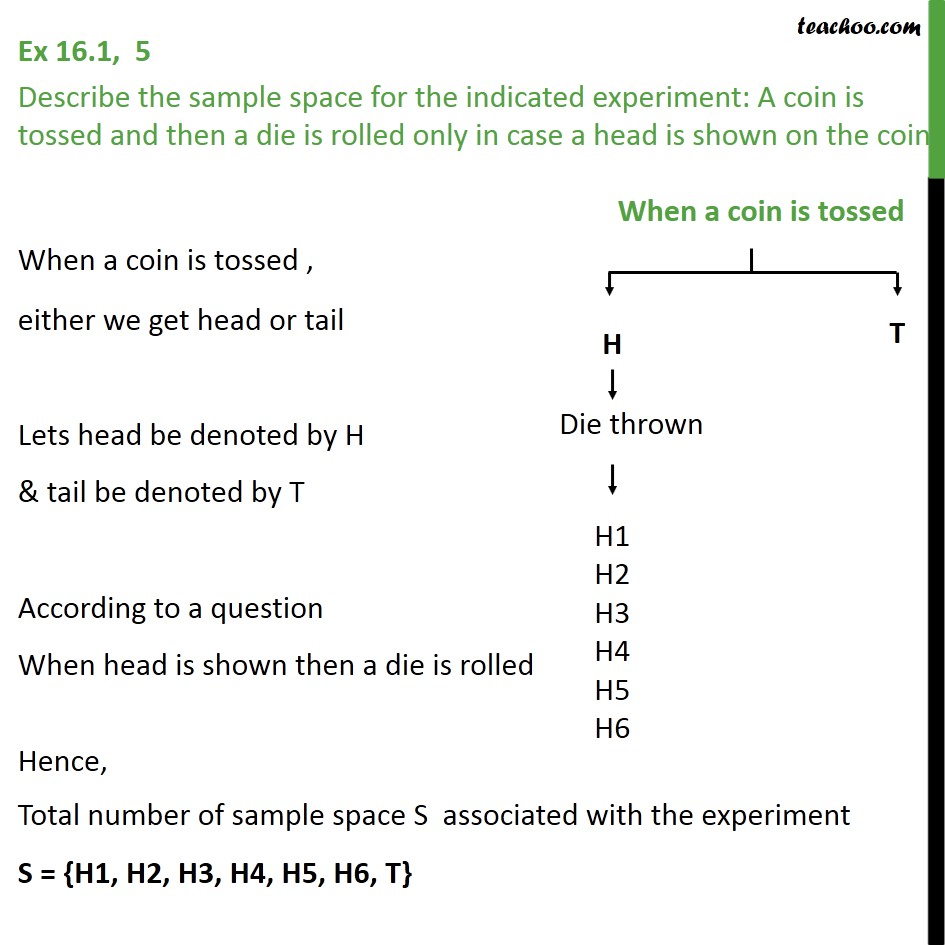1. Chapter 16 Class 11 Probability (Term 2)
2. Serial order wise
3. Ex 16.1

Transcript

Ex 16.1, 5 Describe the sample space for the indicated experiment: A coin is tossed and then a die is rolled only in case a head is shown on the coin. When a coin is tossed , either we get head or tail Lets head be denoted by H & tail be denoted by T According to a question When head is shown then a die is rolled Hence, Total number of sample space S associated with the experiment S = {H1, H2, H3, H4, H5, H6, T}